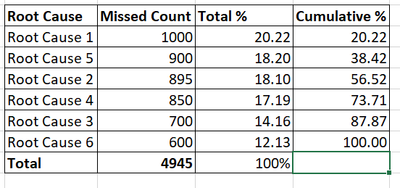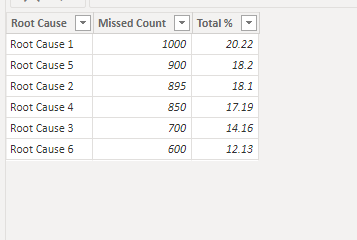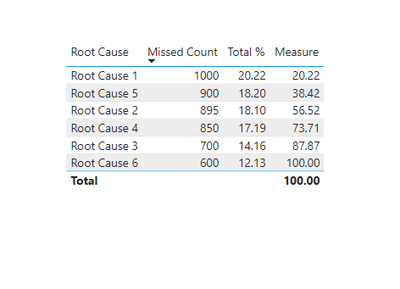cancel
Showing results for
Did you mean:Helper I

## Cumulative total based on sorting order of count total

Hi All,

I have a SQL data source which has multiple columns and many rows, I want to get cumulative total. I have tried several Dax formulas, but nothing is giving me desired result. For my requirement out of many columns i have to use RootCause and MissedCount column and I want to calculate Cumulative total % on descending sort order of MissedCount.

I am able to get cumulative total on sorting order of RootCause using below formula but i want to calculate Cumulative total % based on sorting order of MissedCount.

Cumlative % =
var MissedC=CALCULATE(SUM(Table[MissedCount]),FILTER(ALLSELECTED(Table[RootCause]),ISONORAFTER(Table[RootCause],Max(Table[RootCause]),DESC)))
var totalMissedC=CALCULATE(SUM(Table[MissedCount]),ALLSELECTED(Table))
return DIVIDE(MissedC,totalMissedC)

Expected Cumulative %-Thanks

1 ACCEPTED SOLUTIONCommunity Support

Hi @NSC7 ,

1. below is my test table

Table:2. create measure with below dax formula

``````Measure =
VAR cur_mc =
SELECTEDVALUE ( 'Table'[Missed Count] )
VAR tmp =
FILTER ( ALL ( 'Table' ), [Missed Count] >= cur_mc )
RETURN
SUMX ( tmp, [Total %] )
``````

3. add a table visual with fields and measurePlease refer the attached .pbix file.

Best regards,
Community Support Team_Binbin Yu
If this post helps, then please consider Accept it as the solution to help the other members find it more quickly.Community Support

Hi @NSC7 ,

1. below is my test table

Table:2. create measure with below dax formula

``````Measure =
VAR cur_mc =
SELECTEDVALUE ( 'Table'[Missed Count] )
VAR tmp =
FILTER ( ALL ( 'Table' ), [Missed Count] >= cur_mc )
RETURN
SUMX ( tmp, [Total %] )
``````

3. add a table visual with fields and measurePlease refer the attached .pbix file.

Best regards,
Community Support Team_Binbin Yu
If this post helps, then please consider Accept it as the solution to help the other members find it more quickly.